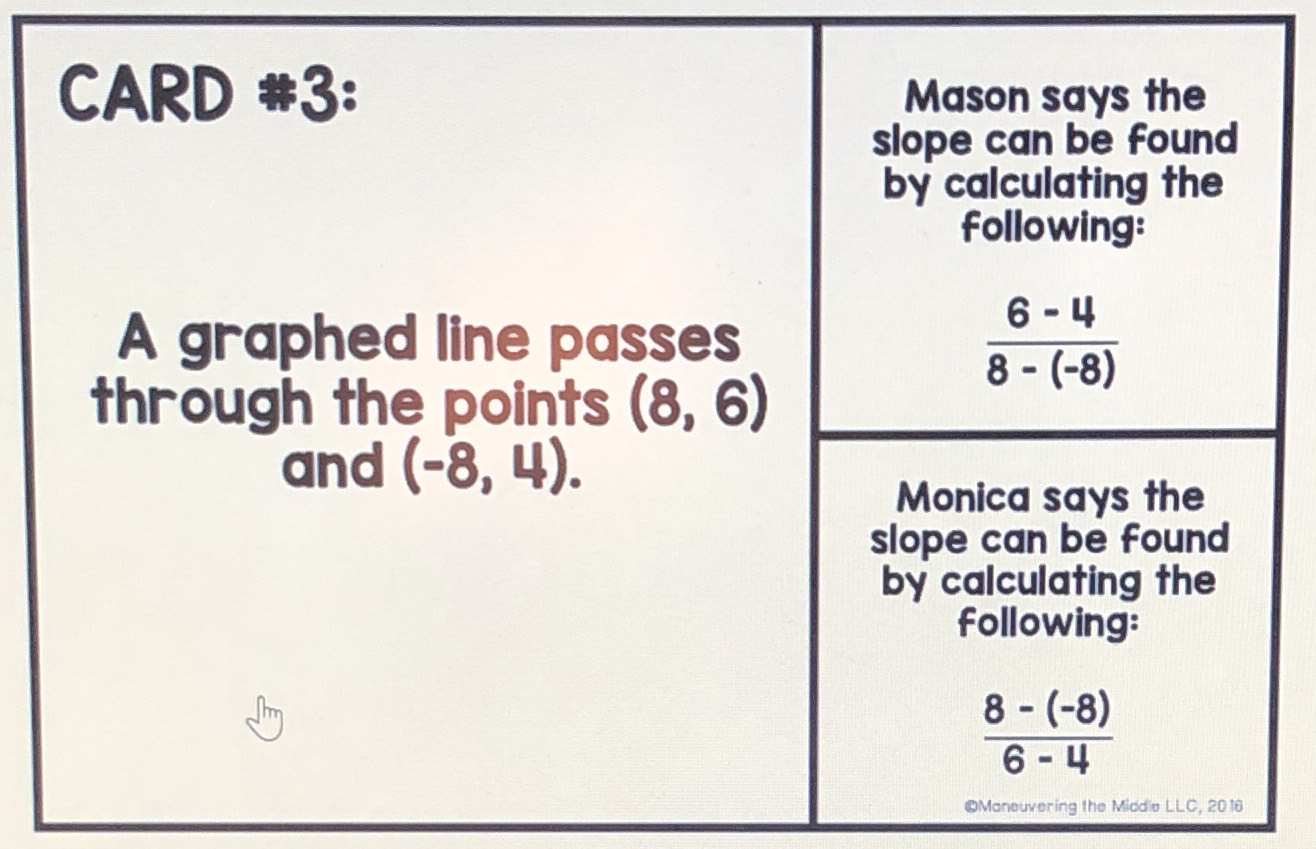### ¿Todavía tienes preguntas de matemáticas?

Pregunte a nuestros tutores expertos
Algebra
PreguntaA graphed line passes through the points $$( 8,6 )$$ and $$( - 8,4 )$$ Mason says the slope can be found by calculating the following: $$\frac { 6 - 4 } { 8 - ( - 8 ) }$$ Monica says the slope can be found by calculating the following: $$\frac { 8 - ( - 8 ) } { 6 - 4 }$$ who is right?